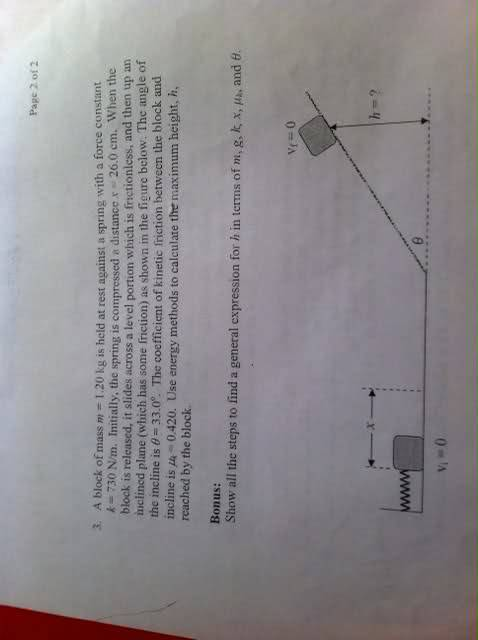# Conservation of energy (Ramp question) - Thanks

## Homework Statement

Here is the question I am having issues with.... Below are my questions regarding it.## The Attempt at a Solution

Now when looking at this, first I would assume I use conservation of momentum.

(K + Ug + Us)i = (K + Ug + Us)f + Ff d

Now for the first part (left side of the equation) my text says the following.

0 + 0 + 1/2Kx^2

I dont get that. So when its at the start, There is no Kinetic energy and no potential evergy, and for the spring energy its just 1/2Kx^2 ?

I dont understand how to figure out the right side of that equation, and mainly finding the height, as outlined in the question.

THanks!!

cepheid
Staff Emeritus
Gold Member

## Homework Statement

Here is the question I am having issues with.... Below are my questions regarding it.## The Attempt at a Solution

Now when looking at this, first I would assume I use conservation of [STRIKE]momentum[/STRIKE] energy.

(K + Ug + Us)i = (K + Ug + Us)f + Ff d

This is correct, once you include my correction above (which I'm sure was just a typo anyway).

Now for the first part (left side of the equation) my text says the following.

0 + 0 + 1/2Kx^2

I dont get that. So when its at the start, There is no Kinetic energy and no potential evergy, and for the spring energy its just 1/2Kx^2 ?

Yes. There is no kinetic energy because the mass is being held *at rest* against the spring. There is no gravitational potential energy, because the mass is at h = 0. The only energy in the system initially is the elastic potential energy stored in the spring, which is indeed given by (1/2)kx2, where x is the displacement of the spring from its equilibrium (unstretched/uncompressed) position. That's really all there is to it. So, if you're still confused, you'll have to elaborate about what specifically is confusing you.

I dont understand how to figure out the right side of that equation, and mainly finding the height, as outlined in the question.

THanks!!

The mass will be released from the spring at the moment that the spring is entirely uncompressed (i.e. x = 0), which means that all of the elastic potential energy is gone. But energy is conserved, which means that all of the elastic potential energy stored must have been converted into kinetic energy of the mass during the release. So, due to conservation of energy, you know how much KE the mass has upon release. Furthermore, the flat portion has no friction, which means that the mass still has this amount of kinetic energy when it reaches the base of the ramp. Therefore, you can use conservation of energy a second time (taking into account work done by friction as well) to determine what height the mass will reach.

cepheid
Staff Emeritus